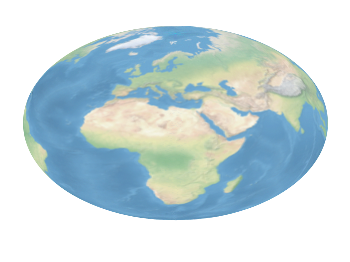# Gnuplotting

Create scientific plots using gnuplot

June 3rd, 2014 | No Comments

Suppose we have a file containing the following data from the plotting data tutorial:

```# absolute_data.txt
# X   Y
1   2
2   3
3   2
4   1
```Fig. 1 Plotting absolute data points. (code to produce this figure, data)

This can be plotted in a straightforward manner and will result in Fig. 1. Now suppose we have the same data points stored as relative coordinates in our data file, resulting in:

```# relative_data.txt
# deltaX deltaY
1   2
1   1
1   -1
1   -1
```

If we want to plot that data in gnuplot we have to keep track of the current position manually by storing its (x,y) value as variables by

```x=0.; y=0.
plot 'relative_data.txt' u (x=x+\$1):(y=y+\$2) w p ls 1
```

Here, we define the starting point to be (0,0) and add to it the values from the first and second column for every line of the data file. By doing so, this results again in Fig. 1. Note, that the addition is always performed first, before the resulting point is plotted which means we get no point at (0,0). Now assume that we also want to add steps going from point to point as shown in Fig. 2. Gnuplot has the `steps` plotting style to achieve this, but we have to be carefully regarding our (x,y) variables.Fig. 2 Plotting relative data points. (code to produce this figure, data)

Every single line of a plotting command is executed after each other which means our (x,y) pair will not be set to (0,0), but to (4,1) at the beginning of the second line of the plotting command. To avoid this we introduce another (a,b) pair for the second line and get finally.

```x=0.; y=0.
a=0.; b=0.
plot 'relative_data.txt' u (x=x+\$1):(y=y+\$2) w steps ls 2,\
''                  u (a=a+\$1):(b=b+\$2) w points ls 1
```

January 31st, 2014 | 3 Comments

And another plot of the world. This time we are dealing with the raster data from Natural Earth. This data is normally available as tif-files. To use them in gnuplot we have to convert them first, then we can create a plot as shown in Fig. 1.Fig. 1 Cross-blended hypsometric tint plot of the world. (code to produce this figure, data)

The conversion is done by the convert_natural_earth script. There the tif-file is first scaled down to the desired resolution using imagemagick. Afterwards it is converted to a text file and reordered for the `splot` command of gnuplot. The text file includes the longitude, latitude and three rgb color values.
You have to invoke the script in the following way.

```\$ ./convert_natural_earth \$RES \$FILE
```

where `\$RES` is the desired resolution in pixel of your gnuplot plot and `\$FILE` the input tif-file.
After finished we can plot the resulting text file simply by

```set datafile separator ','
set size ratio -1
plot 'HYP_50M_SR_W_350px.txt' w rgbimage
```

which results in Fig. 1.Fig. 2 Natural Earth II shaded relief and water plot of the world. (code to produce this figure, data)

The image can also be projected on a 3D figure of the world as shown in Fig. 2. To achieve this the three rgb values have to be summarized in one value and the `rgb variable` line color option has to be chosen together with `pm3d`.

```rgb(r,g,b) = 65536 * int(r) + 256 * int(g) + int(b)
set mapping spherical
set angles degrees
splot 'NE2_50M_SR_W_700px.txt' u 1:2:(1):(rgb(\$3,\$4,\$5)) w pm3d lc rgb variable
```